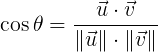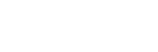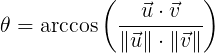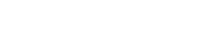# How to check if an object is within an angle of the front of a player’s characters?

Is there any way to check if the object is in the striped area which is within an angle of the front of a player’s character (not camera)?

A simpler concept would be whether there is a way to determine whether an object is in front or behind of the player?

6 Likes

You can get the angle (in radians) between two vectors `a` and `b` with

`angle = math.acos(a:Dot(b)/(a.Magnitude * b.magnitude))`

If the two vectors are perfectly aligned, the angle is `0`. If they’re completely opposite directions, it’s `pi`.

6 Likes

The first thing you want to do is to work out which direction the player’s character is facing:

``````local Facing = Character.HumanoidRootPart.CFrame.LookVector
``````

Then you want to get the direction from your character to the object:

``````local Vector = (Object.Position - Character.HumanoidRootPart.Position).unit
``````

Then you can get the angle between those two vectors using the equation:

Light theme usersDark theme usersWhat this means is that the `cosine` of the angle between vectors `u` and `v` is equal to the dot product of `u` and `v`, divided by the lengths of `u` and `v` multiplied together. The dot on the top of the fraction represents the dot product, and the dot on the bottom represents just a normal multiplication of two numbers.

This can be rewritten as follows:

Light theme usersDark theme usersApplying it to Roblox:

• `arccos` is represented by the `math.acos` function.
• the dot product can be computed using `u:Dot(v)`.
• `u` and `v` are our two vectors we defined at the top which we have called `Facing` and `Vector`.
• the lengths of those two vectors are both `1` (this is how LookVector is defined and because using .unit returns the vector in the same direction but with length `1`).

Therefore, we can get the angle, combining it with the code we wrote above, with the following:

``````local Angle = math.acos(Facing:Dot(Vector) / (1 * 1))
``````

Which simplifies to

``````local Angle = math.acos(Facing:Dot(Vector))
``````

From this point you can just compare the angle and see if it is less than your maximum angle. Note that this angle is in radians, so if you want to compare it to an angle in degrees you’ll need to convert the angle you calculated to degrees using `math.deg`.

117 Likes

Thanks for the detailed explanation! I’ll take a look at it.

1 Like

2 Likes

I wish I could give you an award. Amazing, helped me out ALOT

2 Likes

This was a very good explanation! Thank you very much!

This is a great explanation, but how would you determine if the angle is to the left or right of the HumanoidRootPart’s LookVector?

Hey WhiteKnight, so it’s been 2 years since this question was asked, and I’ve learnt most of the concept in school now, so I think I’m better able to answer this.

Basically, `math.arccos()` returns the acute angle (angle that is less than 90 degrees) between the LookVector and where the camera is facing. To get the direction, you should check if `facing:Dot(Vector) > 0` or `facing:Dot(Vector) < 0`. They indicate either left or right side of the LookVector. That’s all the math theory. Regarding whether more than zero or less than zero is right or left, I think you’ll just have to test in game and see.

1 Like

How would I get the whole angle, instead of just the acute? For example, if I am facing more than 180 degrees away from a point, it would return the bigger angle. This is for my post here: Angle not returning less than 1.5 and more than 4.7